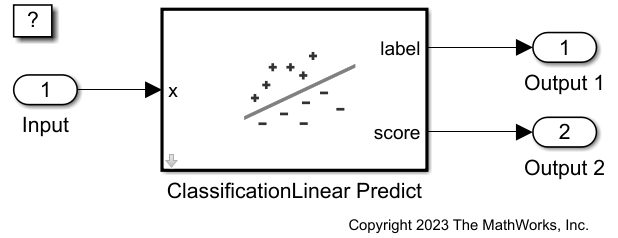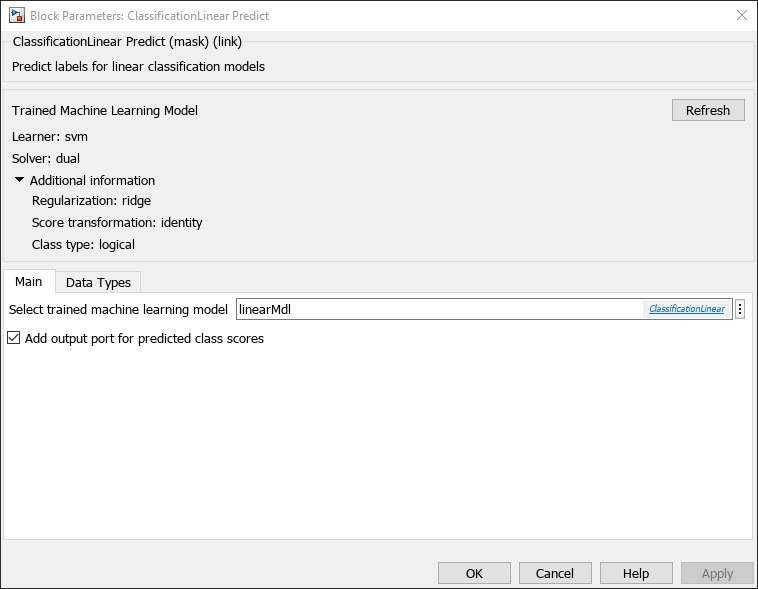# Predict Class Labels Using ClassificationLinear Predict Block

Since R2023a

This example shows how to use the ClassificationLinear Predict block for label prediction in Simulink®. The block accepts an observation (predictor data) and returns the predicted class label and class score for the observation using the trained classification linear model.

### Train Classification Model

Train a model to identify whether a web page is from the Statistics and Machine Learning Toolbox™ documentation based on word frequencies on the page. This type of model is called a bag-of-words model.

Load the `nlp` data set, which contains the predictor matrix `X` and the label vector `Y`. The predictor data `X` is a sparse matrix of word frequencies computed from MathWorks® documentation pages. The labels in `Y` are the names of the toolboxes to which the pages belong.

`load nlpdata`

For more details on the data set, such as the dictionary and corpus, enter `Description`.

Because the observations are arranged by label, shuffle the data set.

```n = size(X,1); rng("default") % For reproducibility shflidx = randperm(n); X = X(shflidx,:); Y = Y(shflidx);```

Identify the labels that correspond to the documentation web pages for Statistics and Machine Learning Toolbox.

`Ystats = Y == "stats";`

Suppose that 90% of the data is observed, and the rest is unseen. Partition the data into present and future samples.

```c = cvpartition(Ystats,Holdout=0.1); idxPrsnt = training(c); idxFtr = test(c); prsntX = X(idxPrsnt,:); prsntY = Ystats(idxPrsnt); ftrX = X(idxFtr,:); ftrY = Ystats(idxFtr);```

Train a linear model using all currently available data.

`linearMdl = fitclinear(prsntX,prsntY);`

`linearMdl` is a `ClassificationLinear` model.

Check the negative and positive class names by using the `ClassNames` property of `linearMdl`.

`linearMdl.ClassNames`
```ans = 2x1 logical array 0 1 ```

The negative class is logical `0`, and the positive class is logical `1`. The logical `1` label indicates that the page is in the Statistics and Machine Learning Toolbox documentation. The output values from the score port of the ClassificationLinear Predict block have the same order. The first and second elements correspond to the negative class and positive class scores, respectively.

This example provides the Simulink model `slexNLPClassificationLinearPredictExample.slx`, which includes the ClassificationLinear Predict block. You can open the Simulink model or create a new model as described in this section.

Open the Simulink model `slexNLPClassificationLinearPredictExample.slx`.

```SimMdlName = "slexNLPClassificationLinearPredictExample"; open_system(SimMdlName)```If you open the Simulink model, then the software runs the code in the `PreLoadFcn` callback function before loading the Simulink model. The `PreLoadFcn` callback function of `slexNLPClassificationLinearPredictExample` includes code to check if your workspace contains the `linearMdl` variable for the trained model. If the workspace does not contain the variable, `PreLoadFcn` loads the sample data, trains the linear model, and creates an input signal for the Simulink model. To view the callback function, in the Setup section on the Modeling tab, click Model Settings and select Model Properties. Then, on the Callbacks tab, select the `PreLoadFcn` callback function in the Model callbacks pane.

To create a new Simulink model, open the Blank Model template and add the ClassificationLinear Predict block. Add the Inport and Outport blocks and connect them to the ClassificationLinear Predict block.

Double-click the ClassificationLinear Predict block to open the Block Parameters dialog box. Specify the Select trained machine learning model parameter as linear`Mdl`, the workspace variable that contains the trained linear model. Click the Refresh button. The dialog box displays the options used to train the linear model `linearMdl` under Trained Machine Learning Model. Select the Add output port for predicted class scores check box to add the second output port score.Create an input signal in the form of a structure array for the Simulink model. The structure array must contain these fields:

• `time` — The points in time at which the observations enter the model. In this example, the duration includes the integers from 0 through `nftrX - 1`, where `nftrX` is the number of samples in the input data. The orientation must correspond to the observations in the predictor data. So, in this case, `time` must be a column vector.

• `signals` — A 1-by-1 structure array describing the input data and containing the fields `values` and `dimensions`, where `values` is a matrix of predictor data, and `dimensions` is the number of predictor variables.

Create an appropriate structure array for the future samples in the sparse matrix `ftrX` after converting the matrix to full storage organization by using the `full` function.

```[nftrX,p] = size(ftrX); nlp.time = (1:nftrX)' - 1; nlp.signals(1).values = full(ftrX); nlp.signals(1).dimensions = p;```

To import signal data from the workspace:

• Open the Configuration Parameters dialog box. On the Modeling tab, click Model Settings.

• In the Data Import/Export pane, select the Input check box and enter `nlp` in the adjacent text box.

• In the Solver pane, under Simulation time, set Stop time to `nlp.time(end)`. Under Solver selection, set Type to `Fixed-step`, and set Solver to `discrete (no continuous states)`. Under Solver details, set `Fixed-step size` to 1. These settings enable the model to run the simulation for each sample in `nlp`.

`sim(SimMdlName);`